Scattering of X-rays by a Single Electron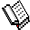Course Material Index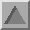Section Index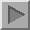Next Page

Scattering of X-rays by a Single Electron

It is useful to consider the scattering of X-rays by an electron to be a fundamental scattering process (later we will also consider the scattering of neutrons). The unit of X-ray energy is termed an X-ray photon and can be imagined as a finite travelling wave of electromagnetic displacement. Many of the diagrams we will use will be highly schematic (and should not always be taken too literally); the first diagram falls into this class, illustrating the basic features of the electric displacement of an X-ray photon: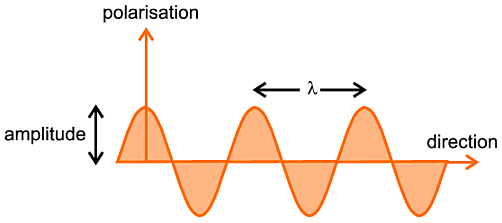The terms used are important: amplitude (the maximum displacement), wavelength denoted by λ (the distance between successive maxima), direction of propagation of the X-ray photon (horizontal from left to right as it appears on your screen), and polarisation (the direction of electric displacement) which appears vertical on your screen.

An X-ray can be scattered by an electron. This can be perceived by the change in direction of the X-ray after it has "passed" the electron (so to speak), as illustrated in the following schematic diagram: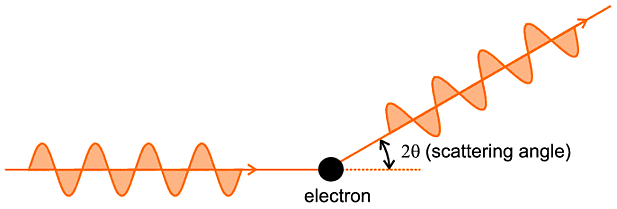Again this diagram is somewhat naive (and perhaps questionable on true quantum mechanical grounds) but it gets the essential ideas over. It is not so important to understand exactly what goes on as the X-ray interferes with and is scattered by the electron, but the net end result is important: both the direction and polarisation of the X-ray photon have been altered by an angle 2θ. There is nothing to worry about by the use of 2θ as the angle of scattering (rather than, say, θ this is simply a matter of convention at this stage. We might also point out that the X-ray photon also suffers a phase shift of 180 degrees (π radians), but this is not important either at this stage.

This whole process is often summarised by an important vector diagram and accompanying vector equation, though you do not really need to understand much about vector algebra to follow these: since a vector is something which has both magnitude and direction we can represent two items, the absolute momentum of the X-ray photon and its direction of propagation, by a vector. One other fact you should accept is that the momentum of a wave is proportional to the inverse of its wavelength (a result discovered earlier in the 20th century by Planck); so we make the length of the vector equal to 1/λ and the direction of the vector the same as the propagation direction of the X-ray. Then we represent the scattering event by a vector diagram, which follows, in which so represents the direction and momentum of the incident X-ray photon (i.e. before it is scattered) and s represents the direction and momentum of the X-ray photon after being scattered. An important aspect of diffraction is that we only consider elastic scattering; by that we mean that there is no loss of X-ray photon energy through scattering so that the energy (and absolute momentum) of the X-ray photon remains unchanged. This is why the length of s in the diagram (1/λ) has been made the same as the length of so. Finally the vector S, which bridges s and so, represents the net change as a result of the scattering process and is an important item, often referred to as the scattering vector S; its length is equal to 2 sinθ / λ (you might like to try proving this from the properties of the isosceles triangle in the diagram).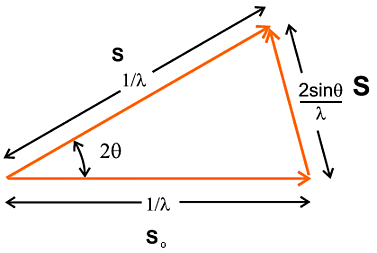The vector equation that summarises the above figure is:
s = so + S
which basically re-iterates that the scattered wave (s) is the result of the incident wave (so) being subjected to the scattering process (+ S).Course Material IndexSection IndexNext Page
 © Copyright 1997-2006.  Birkbeck College, University of London. Author(s): Paul Barnes Simon Jacques Martin Vickers